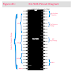Here, in this article, we are going to see ICL7106 and IC L7107 Pinout Diagram, Pin Configuration, Features, etc. The ICL7106 and ICL7107 are integrated circuit (IC) chips developed by Intersil (now part of Renesas Electronics) that are commonly used for digital voltmeter and panel meter applications. These ICs are designed to simplify the construction of digital multimeters and other measurement devices.

ICL7106 and ICL7107 are functionally similar, with the main difference being their display modes. Both ICs are 3½ digit analog-to-digital converters (ADCs) that can convert an analog input voltage into a digital representation. This allows you to measure and display voltages accurately using a microcontroller or other digital display components.

## ICL7106 Pinout Diagram

Here, you can see the pinout diagram of ICL7106.

Here are the functions of each pin described below,

Pin no. 1 is the Supply voltage (+) Pin that is used to provide the operating voltage for the IC

Pin 2,3,4,5,6,7 and 8 are output pins for the 7-Segment 4th digit(1's) display. These pins are connected to the 7-segment display for displaying the one digit of the output value.

Pins 9,10,11,12,13,14 and 25 are output pins for a 7-segment 3rd digit(10's) display. These pins are connected to the 7-segment  for displaying the tenth-digit

Pin 15,16,17,18,22,23 and 24 are output pins for a 7-segment 2nd digit(100's) display. These pins are connected to the 7-segment  for displaying the hundredth-digit

Pin 19 and 20 are output pins for a 7-segment 1st digit(1000's) display. These pins are connected to the 7-segment  for displaying the thousandth digit

Pin no. 21 is Ground. It is connected to the ground of the system.

Pin no. 26 is Supply voltage (-). It is used to provide the negative supply voltage to the IC.

Pin no. 27 is the Signal integrate pin. It is to be connected to a capacitor.

Pin 28 and 29 are used for Auto Zero and buffer. These are to be connected to the capacitor and resistor network.

Pin 30 and 31 Input High and Input Low Pins. The analog voltage that has to be measured is to be connected here.

Pin 32 is a Common Pin. It is to be connected to the ground.

Pin 33 and 34 are Cref+ and Cref- respectively. A capacitor is to be connected across these pins for neutralizing fluctuations in reference voltage

Pin 35 and 36 are Reference High and Reference Low respectively. Either can supply reference voltage or use internal reference voltage.

Pin 37 is the Test pin. It is generally not used during operation.

Pin 38, 39 and 40 are OSC1, OSC2, OSC3 respectively. These are used to build an RC network top-set oscillator frequency.

## ICL7106 Features

2. Designed for driving common-cathode LED displays.
3. Requires external multiplexing to drive seven-segment displays.
4. Suitable for applications where the display drive circuitry is not integrated into the IC.
5. Commonly used in handheld digital multimeters and other measurement devices.

## ICL7107 Pinout Diagram

Here, you can see the Pinout diagram of ICL7107.

As you can see the Pinout diagram of ICL7107 is the same as the ICL7106. So the function of each pin is also the same that is already described above.

## ICL7107 Features

ICL7106 and ICL7107 Pinout Diagram, Features, DatasheetReviewed by Author on September 08, 2023 Rating: 5